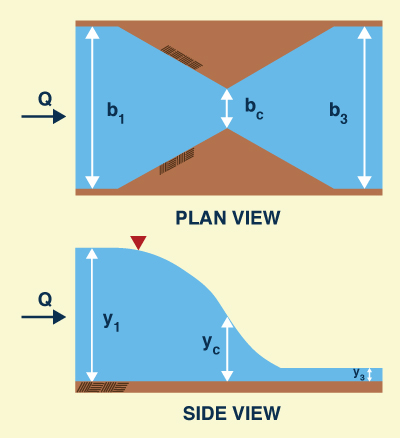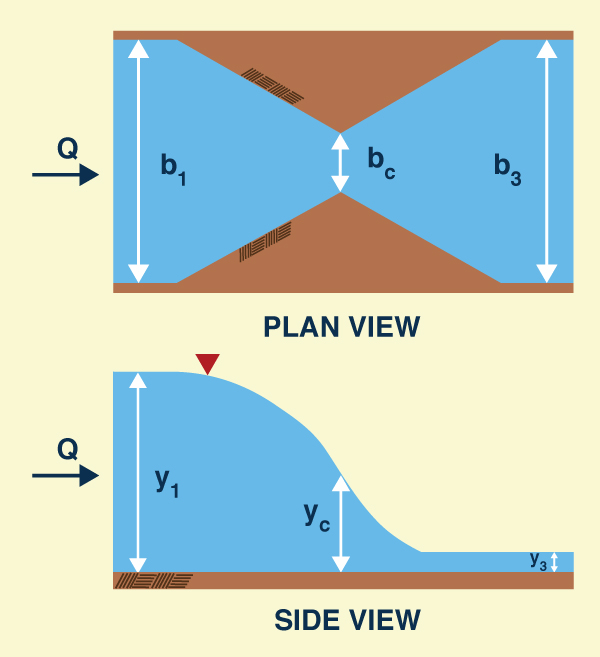The Limiting Contraction RatioRevisited Nicole R. Nuccitelli and Victor M. Ponce April 2014

 ABSTRACT Henderson's formulations of the energy-based and momentum-based limiting contraction ratios are reviewed (Henderson 1966). Henderson's explicit energy-based equation is found to be correct, however, his implicit momentum-based equation is found to be incorrect. A new explicit momentum-based equation is derived, rendering the implicit formulation unnecessary. An online calculator enables the calculation of the limiting contraction ratio for both energy and momentum formulations.

1.  THE LIMITING CONTRACTION RATIO

The limiting contraction ratio σ is the ratio of contraction width bc to upstream channel width b1 (σ = bc /b1), or, alternatively, downstream channel width b3 (σ = bc /b3), that produces critical flow at the contraction in a rectangular channel. The ratio has an upper limit σ = 1 when the upstream or downstream flow is critical.

According to Henderson (1966), the ratio σ may be calculated in two ways: (1) using an energy balance between the upstream and critical contraction sections, or (2) using a momentum balance between the critical contraction and downstream sections. Henderson has argued that the momentum balance is more likely to be correct because it does not depend on an assumption of energy conservation. However, Henderson's implicit formulation of the momentum-based limiting contraction ratio appears to be incorrect. These propositions are now substantiated.

2.  ENERGY-BASED EQUATION FOR LIMITING CONTRACTION RATIO

The energy-based equation is derived by equating the specific energy at the upstream flow section (Section 1) with the specific energy at critical flow at the contraction (Section 2, or Section c) (Fig. 1):

 E1  =  E2  =  Ec (1)

The specific energy of the upstream flow is:

 v12    E1  =  y1  +  _______                        2 g (2)

The Froude number at the upstream section is defined as follows:

 v12    F12  =  _______               g y1 (3)

Thus, Eq. 2 reduces to:

 F12    E1  =  y1 [ 1  +  ______ ]                              2 (4)

By definition, the critical depth is:

 F12    yc  =  (2/3) E1  =  (2/3) y1 [ 1  +  ______ ]                                                        2 (5)

Thus:

 yc                              F12    _____  =  (2/3) [ 1  +  ______ ]   y1                               2 (6)

Or, alternatively:

 yc                                  _____  =  (1/3) [ 2  +  F12 ]   y1 (7)

From continuity:

 v1 y1 b1  =  vc yc bc (8)

The limiting contraction ratio σ is:

 bc             v1 y1 σ  =  _______  =  ________             b1             vc yc (9)

Or:

 v1 σ  =  _____________            vc (yc /y1 ) (10)

Or:

 v1 σ  =  ___________________            (g yc)1/2 (yc /y1 ) (11)

Or:

 v1 σ  =  ______________________            (g y1)1/2 (yc /y1 )3/2 (12)

Replacing Eq. 3 in Eq. 12:

 F1 σ  =  ______________             (yc /y1 )3/2 (13)

Replacing Eq. 7 in Eq. 13:

 F1 σ  =  ________________________            (1/3)3/2 (2  +  F12) 3/2 (14)

Simplifying Eq. 14:

 33/2 F1 σ  =  _________________            (2  +  F12) 3/2 (15)

Equation 15 is the energy-based limiting contraction ratio.

Squaring Eq. 15:

 33 F12 σ2  =  ________________               (2  +  F12) 3 (16)

Equation 16 is the same as that presented by Henderson for the limiting contraction ratio (op. cit., Eq. 7-35, p. 267).Fig. 1  Definition sketch.

3.  MOMENTUM-BASED EQUATION FOR LIMITING CONTRACTION RATIO

The momentum-based equation is derived by equating the specific force at critical flow at the contraction (Section 2, or Section c) with the specific force at the downstream flow section (Section 3).

 M3  =  M2  =  Mc (17)

The specific force at the downstream flow section is:

 q32             y32    M3  =  _______  +  _______              g y3             2 (18)

Replacing the discharge Q:

 Q2                y32    M3  =  ___________  +  ______              g y3 b32             2 (19)

From continuity:

 Q = v3 y3 b3  =  vc yc bc (20)

Thus:

 v32 y32            y32    M3  =  __________  +  ______                g y3                2 (21)

The Froude number at the downstream section is defined as follows:

 v32    F32  =  _______               g y3 (22)

Thus, Eq. 21 reduces to:

 1                 M3  =  y32 ( ____  +  F32 )                      2 (23)

The specific force at the contraction is:

 qc2             yc2    Mc  =  _______  +  _______              g yc             2 (24)

Replacing the discharge Q:

 Q2                yc2    Mc  =  ___________  +  ______              g yc bc2             2 (25)

From continuity:

 v32 y32 b32            yc2    Mc  =  _______________  +  ______                  g yc bc2                2 (26)

Or:

 v32 y33 b32            yc2    Mc  =  _______________  +  ______                g yc bc2 y3              2 (27)

Replacing Eq. 22 in Eq. 27:

 F32 y33            yc2    Mc  =  ___________  +  ______                σ2 yc               2 (28)

By definition, the critical depth is:

 q2                   yc  =  ( ____ ) 1/3               g (29)

Or:

 Q2                   yc  =  ( ________ ) 1/3                bc2g (30)

From continuity:

 v32 y32 b32                    yc  =  ( _____________ ) 1/3                    bc2g (31)

Or:

 v32 y33 b32                    yc  =  ( _____________ ) 1/3                  bc2g y3 (32)

Replacing Eq. 22 in Eq. 32:

 F32 y33                    yc  =  ( _________ ) 1/3                  σ2 (33)

Replacing Eq. 33 in Eq. 28:

 F32 y32 σ2/3                     F32 y33 Mc  =  ______________  +  (1/2) ( _________ )2/3                σ2 F32/3                            σ2 (34)

Reducing terms:

 F34/3 Mc  =  (3/2) ( ________ ) y32                          σ4/3 (35)

Equating Eqs. 23 and 35:

 1                                   F34/3 ( ___  +  F32 )  =  (3/2) ( ________ )     2                                    σ4/3 (36)

Reducing:

 33/4 F3 σ  =  _____________________             ( 1  +  2 F32 ) 3/4 (37)

Equation 37 is the explicit formulation of the momentum-based limiting contraction ratio.

4.  IMPLICIT MOMENTUM-BASED FORMULATION

To derive an implicit formulation, Eq. 36 is expressed as follows:

 3 F34/3 σ4/3  =  _______________                 1  +  2 F32 (38)

Or:

 (3/σ) F34/3 σ1/3  =  _______________                 1  +  2 F32 (39)

Reducing:

 (3/σ)3 F34 σ  =  _________________             (1  +  2 F32)3 (40)

Equation 40 differs slightly from Henderson's Eq. 7-36, page 267, repeated here:

 (2 + 1/σ)3 F34 σ  =  __________________             (1  +  2 F32)3 (41)

Thus, Henderson's implicit equation for the momentum-based limiting contraction ratio (Eq. 41) appears to be incorrect.

5.  SUMMARY

Two explicit equations for the limiting contraction ratio, the first based on an energy balance, and the second based on a momentum balance, are derived following Henderson (1966). An implicit equation based on momentum balance is also derived, to parallel Henderson's treatment.

The explicit energy-based equation, which is shown to be same as Henderson's, is:

 33/2 F1 σ  =  _________________            (2  +  F12) 3/2 (15)

The explicit momentum-based equation, not presented by Henderson, is:

 33/4 F3 σ  =  __________________            (1  +  2 F32) 3/4 (37)

Note the great resemblance between Eq. 15 (energy-based) and Eq. 37 (momentum-based).

The implicit momentum-based equation derived here (Eq. 40) differs slightly from that of Henderson (Eq. 41), suggesting that the latter is incorrect.

The online calculator ONLINE_LIMITING_CONTRACTION calculates the energy-based and momentum-based limiting contraction ratios for a pair of Froude numbers F1 and F3. Additionally, the online calculator ONLINE_LIMITING_CONTRACTION_SET calculates the set of energy-based and momentum-based limiting contraction ratios for a given range of Froude numbers and Froude number interval.

REFERENCES

Henderson, F. M., 1966. Open channel flow. MacMillan Publishing Co., Inc., New York.